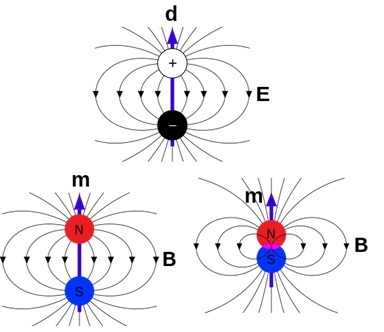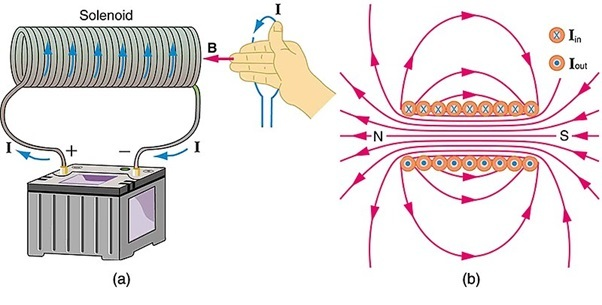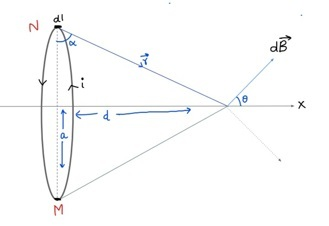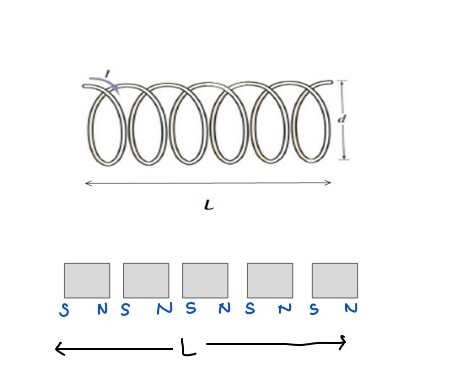# Bar Magnet As An Equivalent Solenoid

## Introduction

Bar magnet is an equivalent to a Solenoid. We all have seen Bar magnets and played with them in our childhood. It has astounding properties of attracting certain kinds of materials. For example, it attracts iron but shows neutral behaviour towards plastic. Bar Magnets are made of some special kind of materials called ferromagnetic materials and they have permanent magnetic behaviour.

Magnets are very useful in today’s technological world. Many electronic devices such as speakers, hard drives, and TV use magnets. We know, that electric current also can produce magnetic fields. Can we produce something like a bar magnet from an electric current? The answer is yes. In the next sections, we will see how a coil can behave like a bar magnet in certain configurations. It produces magnetic fields like a bar magnet but has certain differences too. Here first we will look into bar magnet and solenoid separately, then we can derive the equation which shows that they produce a similar kind of magnetic field.

## What is a Bar Magnet?

A bar magnet is a piece of matter having magnetic poles on both of its ends. It is rectangular as shown in the figure.

If we hang it freely from the mid-point, one end will point towards the geographic north and the other end towards geographic south. One is called the north pole and the other one is called the south pole. Usually, these kinds of magnets are made of Iron, Aluminium, Nickel, etc. We can compare this to a situation in electrostatics, where two charges form an electric dipole. Here the bar magnet works as a magnetic dipole.

## Magnetic dipole

Suppose m denotes positive magnetic charge (N pole) and -m denotes negative magnetic charge (S pole) and they are d distance apart. Then

$$\mathrm{Magnetic\:dipole\:\:\: \overrightarrow{\mu}=md}$$

And the direction is from -m to +m or S to N.Maschen, Em dipoles, CC0 1.0

We can calculate the intensity of the magnetic field due to this and in the equatorial position the intensity is given by

$$\mathrm{\overrightarrow{B}=\frac{\mu_0}{4\pi}\frac{2\overrightarrow{\mu}}{r^2}………………. (i)}$$

## What is Solenoid?

The solenoid is a long wire which is wound in the shape of a coil. Its length is very large in comparison to its diameter.

## What is the Use of Solenoid?

It can be used as an electromagnet. They can be used in Antennas, inductors, and circuit breakers also.OpenStax, Openstax college-physics 22.40 solenoid-Bfield, CC BY 4.0

Magnetic due to solenoid can be calculated using ampere’s law.

If n=no of turns per unit length and i is the current flowing in it.

Then

$$\mathrm{\overrightarrow{B}=\mu_0 n i(inside)}$$

## Derivation of Bar Magnet as an Equivalent Solenoid

First, we will calculate the magnetic field due to the current-carrying loop on an equatorial point. We take a circular loop of radius ‘a’ and the current in the wire is let’s say i.Suppose there is point P at distance d from the center of the loop. We want to compute here the magnetic field due to the circular current-carrying loop.

Let us take a small length element dl and compute the field due to it at P. Suppose the vector joining N and P is $\mathrm{NP=\overrightarrow{r}}$

We use biot-savart's law

$$\mathrm{\overrightarrow{dB}=\frac{\mu_0}{4\pi}\frac{i\overrightarrow{dl}\times \overrightarrow{r}}{r^3}}$$

We can in the figure that $\mathrm{\overrightarrow{dl}}$ is perpendicular to the plane of the figure and $\mathrm{\overrightarrow{dl}\times \overrightarrow{r}}$ will be on the plane.

$$\mathrm{I.e.\:\:\:\overrightarrow{dl}\times \overrightarrow{r}=dl \:r}$$

$$\mathrm{dB =\frac{\mu_0}{4\pi}\frac{idl}{r^2}}$$

we can use Pythagoras theorem in the figure and calculate that

$$\mathrm{r^2= a^2+d^2}$$Hence

$$\mathrm{dB =\frac{\mu_0}{4\pi}\frac{idl}{(a^2+d^2)}}$$

If we take the same element at M, we can see that it will be resolved in horizontal and vertical components. Where the vertical component of M will cancel the component of N. Hence only the Horizontal component will contribute. If we extend this logic to small elements of the whole loop then the only contribution will be from horizontal components.

The horizontal component of an element will be

$$\mathrm{dB_x = dB\:cosθ}$$

Hence net field due to the circular loop will be

$$\mathrm{B=\int dBcosθ=\int \frac{\mu_0}{4\pi}\frac{idl}{(a^2+d^2)}cosθ}$$

From figure

$$\mathrm{cosθ=\frac{a}{\sqrt{a^2+d^2}}}$$

$$\mathrm{B=\int \frac{\mu_0}{4\pi}\frac{idl}{(a^2+d^2)}\frac{a}{\sqrt{a^2+d^2}}=\frac{\mu_0}{4\pi}\frac{i}{(a^2+d^2)}\frac{a}{\sqrt{a^2+d^2}}\int dl=\frac{\mu_0}{4\pi}\frac{i}{(a^2+d^2)}\frac{a}{\sqrt{a^2+d^2}}2\pi a}$$

Finally,

we get

$$\mathrm{B =\frac{\mu_0 i a^2}{2(a^2+d^2 )^{3/2}}……………………. (ii)}$$

## Solenoid as a Magnet Bar

In equation (ii) if we take a case where the field point P is far away from the coil (d>>a)

Then

$$\mathrm{a^2+d^2\thickapprox d^2}$$

Hence

$$\mathrm{B =\frac{\mu_0\:i\: a^2}{2d^3}=\frac{2\mu_0 (\Pi a^2)}{2\Pi 2d^3}}$$

We know the magnetic moment of current-carrying wire is $\mathrm{\overrightarrow{\mu}=iA=i\pi a^2}$

It gives us

$$\mathrm{\overrightarrow{B}=\frac{\mu_0}{4\pi }\frac{2\overrightarrow{\mu}}{d^2}}$$

This equation looks familiar. It is the same as equation (i) which is the magnetic field due to a bar magnet. Hence, we can see that solenoids which consist of many such current-carrying circular loops can work as a bar magnet.

## Difference between a Bar Magnet and a Solenoid

These are major differences between bar magnets and solenoids.

• A solenoid can work as a magnet only if the current is flowing in it, however, the bar magnet is a permanent magnet.

• The strength of the magnetic field of a bar magnet is fixed, so we can't alter it easily. But we can change the magnetic field of the solenoid by increasing or decreasing the current.

• The polarity of the bar magnet is fixed, but in the case of the solenoid, it can be altered.

## Similarities between a Bar Magnet and a Solenoid

These are similarities between bar magnet and solenoid.

• The magnetic field at the equatorial point is the same for bar magnet and solenoid.

• Both experience torque when placed in an external field

• Both have magnetic properties like attraction and repulsion.

• The direction of the field line is the same in both of them.

## How Does a Solenoid Behave Like a Magnet?If we see a solenoid closely it is similar to a combination of many circular loops (as shown in the figure). If current i is flowing in a loop, then each turn will have a magnetic moment equal to iA. Each turn can be thought of as a small magnetic dipole of a magnetic moment μ = md.

If we take the distance d so close that the N pole of one touch the S pole of the other and so on. Then the whole configuration can be replaced by just a bar magnet having one north and one south pole.

## Conclusion

Current carrying wire produces a magnetic field at an equatorial point which can be equal to the current produced by a magnetic dipole. For this, the distance between the point at which we are computing the field should be very large in comparison to the radius of the coil. The solenoid can be modelled as several parallel coils within a very close distance. Hence it can work as a bar magnet. They are similar since they produce a similar magnetic field but they are different since one is a permanent magnet and another one is an electromagnet.

## FAQs

Q1. What is the Difference between the electric and magnetic dipole?

Ans. Electric dipoles consist of two entities namely - positive and negative charges which have their existence. But there cannot be an independent north or south pole. They are always in pairs.

Q2. How many types of magnets are there?

Ans. Generally, there are three types of magnets - Permanent, Temporary, and Electromagnet.

Q3. What is the value of the magnetic field produced by the solenoid outside of it?

Ans. Value of magnetic field outside of solenoid is zero, since the current is zero there.

Q4. Can we use ampere’s law in the computing field due to circular wire on an equatorial point?

Ans. No, it can be applied to highly symmetrical cases only.

Q5. How does the magnetic field arise from a bar magnet without any current?

Ans. At the microscopic level moving electrons create current at the atomic level. In magnets, these small current loops are parallel arranged, so they don’t cancel out each other.

Updated on: 28-Apr-2023

1K+ Views